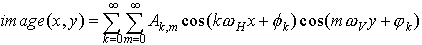EECS20N: Signals and Systems

# Fourier series approximations to images

Images are invariably finite signals. Given any image, it is possible to construct a periodic image by just tiling a plane with the image. Thus, there is again a close relationship between a periodic image and a finite one.

We have seen sinusoidal images, so it follows that it ought to be possible to construct a Fourier series representation of an image. The only hard part is that images have a two-dimensional domain, and thus are finite in two distinct dimensions. The sinusoidal images that we saw correspondingly could have a vertical frequency, a horizontal frequency, or both.

Suppose that the domain of an image is [a, b] × [c, d] ⊂ Reals × Reals. Let pH = b − a, and pV = d− c represent the horizontal and vertical "periods" for the equivalent periodic image. For constructing a Fourier series representation, we can define the horizontal and vertical fundamental frequencies as follows:

ω H = 2π /pH
ω V = 2π /pV

The Fourier series representation of Image: [a, b] × [c, d] → Intensity isFor convenience, we have included the constant term A0,0 in the summation, so we assume that Φ 0 = φ 0 = 0 (recall that cos(0) = 1).